Want to share your content on R-bloggers? click here if you have a blog, or here if you don't.

Although you will learn in this article how to create inforgraphics in R, I will be honest: I don’t like meaningless, busy, and unactionable infographics. Then why did I write this article? A couple of reasons:

1. I love the message of this chart
2. I wanted to see what’s possible in R## The message

Gary Keller, in his book The One Thing, emphasized identifying the one thing in your life or work that is central to your success. If you keep doing that one thing repeatedly, you will overcome obstacles and be successful. He gives many examples of how the singular focus has helped him and others. He argues that we spend too much time on too many things (priorities?), and we set ourselves to underachieve. Nothing can stop us from succeeding if everyday we just identify that one thing, and then tirelessly work on that one thing. Keller provided a “sticky” metaphor to explain this.

## An Example

One particular example stood up for me. How one small initial thing and momentum can help you reach your goals. He talks about dominoes and momentum. You already know the expression “fall like dominoes” i.e. if we line up dominoes and push one down, the rest also come down pushing the next one. This example, however, uses geometric series of the form 2, 2*1.5, 3*1.5, 4*1.5, …., n*1.5.

We start with a domino 2in tall, second one is 2*1.5 = 3in, third is 3*1.5 = 4.5in, fourth is 4*1.5 = 6in, fifth is 5*1.5 = 7.5in, and so on. Although these are different sizes and we start really small, just like regular dominoes, these will also push the next one down. You may have seen this in a Prudential commercial:

(for fun watch this video and this one)

Now you may ask: “What’s so special about this?” Well, the theory supports that like regular dominoes, these dominoes could keep pushing the next ones down. Even the small, 2in domino can bring down the tallest of dominoes. You keep going on and,

• with the 15th domino you will reach the height of one of the tallest dinosaurs, Argentinosaurus (16m tall)
• with the 18th domino you will reach at the top of the statue of liberty (46m)
• with the 40th domino you be in the space station (370km)
• and by 57th, you will reach the moon (370,000km)

People use the expression “reach for the moon” meaning try to achieve very difficult tasks, in almost a defeating tone; however, this example empowers us to think that it IS indeed possible for a small domino to build up to reach the moon.

It might be easy for some people to sustain with whatever current knowledge they have, but we know that in the knowledge economy we must continuously improve and learn. I heard this recently from Mike Rayburn: “coasting only happens downhill.” Although it is easy to coast at a job, it will only bring us down. Another difficult, but invigorating approach is to become a “virtuoso“: mastery in the chosen field.virtuoso
noun
a person who has a masterly or dazzling skill or technique in any field of activity

Reaching for the moon is of course extremely difficult and improbable for most of us, but the metaphor is powerful. We start with one step small step, we repeat that step with increased intensity and momentum, and we can achieve our goals. With focus, efforts and momentum, it is possible to achieve even the most improbable goals. Find your one thing that will make you successful and repeat it everyday in increasing order.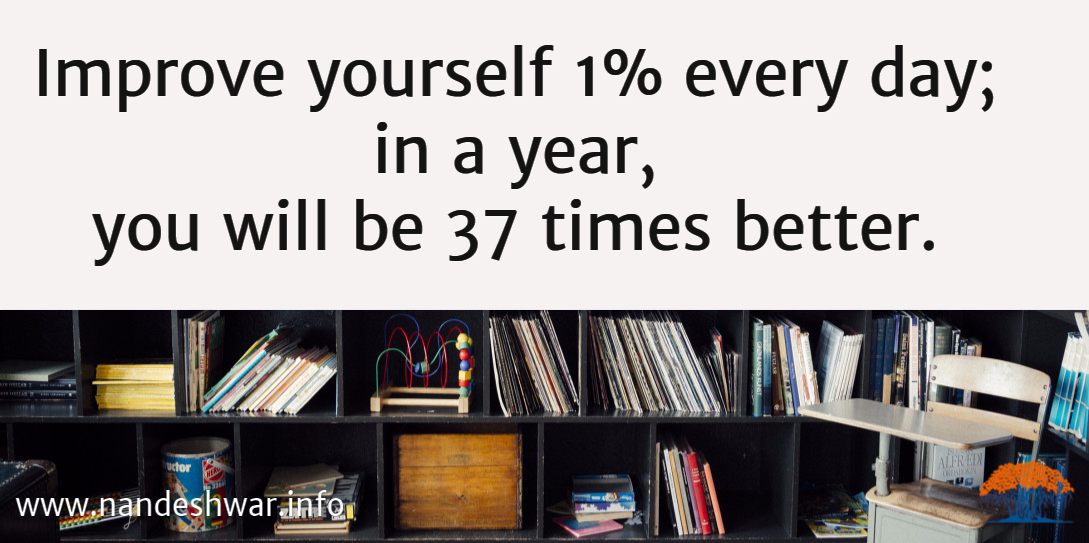## Recipe for Infographics in R

### Ingredients

Now I feel better. I justified myself to replicate the above example in R. After I justified myself, I searched for some basics and found some fantastic threads on stackoverflow on using images in `R` and `ggplot2`.

### Hint

It was hardly obvious to me that inforgraphics in statistics are called pictograms. Remember this when you search for information on infographics in R

After knowing that it was possible to create infographics in R, I searched for some vector art. I found them on vecteezy.com and vector.me.

### Not lying

Edward Tufte, in his book The Visual Display of Quantitative Information, famously described how graphic designers (or let’s say data communicators) “lie” with data, especially when the objects they plot are hardly in true proportions. My challenge was thus to avoid lying and still communicate the message.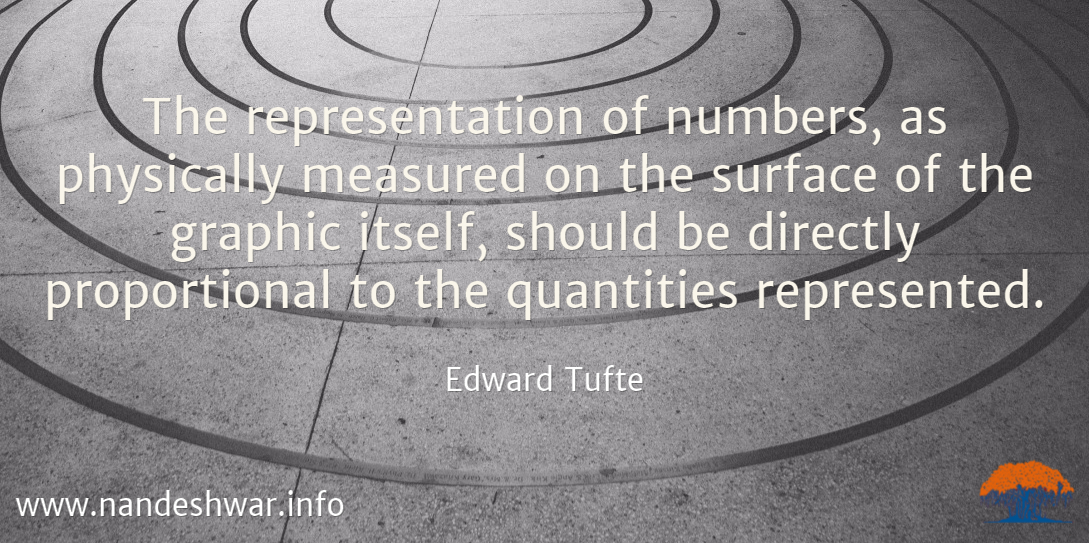### R code

Now to the fun part! Getting our hands dirty in `R` when not pulling our hair dealing with `R`.

#### Step 1:Load my favorite libraries

```library('ggplot2') library('scales') # for number formating library('png') # to read png files library('grid') # for expanding the plot library('Cairo') # for high quality plots and anti-aliasing library('plyr') # for easy data manipulation```

#### Step 2: Generate data and the base plot

```dominoes <- data.frame(n = 1:58, height = 0.051 *1.5^(0:57)) # 2inch is 0.051 meters base_plot <- qplot(x = n, y = height, data = dominoes, geom = "line") #+ scale_y_sqrt() base_plot <- base_plot + labs(x = "Sequence Number", y = "Height/Distance\n(meters)") + theme(axis.ticks = element_blank(), panel.background = element_rect(fill = "white", colour = "white"), legend.position = "none") base_plot <- base_plot + theme(axis.title.y = element_text(angle = 0), axis.text = element_text(size = 18), axis.title = element_text(size = 20)) base_plot <- base_plot + theme(plot.margin = unit(c(1,1,18,1), "lines")) + scale_y_continuous(labels = comma) base_plot```

### Note

The argument `plot.margin`. I increased the height of the plot by supplying the parameter `unit(c(1,1,18,1), "lines")`

We get this plot:#### Step 3: Read all the vector arts in a Grob form

```domino_img <- readPNG("domino.png") domino_grob <- rasterGrob(domino_img, interpolate = TRUE)   eiffel_tower_img <- readPNG("eiffel-tower.png") eiffel_tower_grob <- rasterGrob(eiffel_tower_img, interpolate = TRUE)   pisa_img <- readPNG("pisa-tower.png") pisa_grob <- rasterGrob(pisa_img, interpolate = TRUE)   liberty_img <- readPNG("statue-of-liberty.png") libery_grob <- rasterGrob(liberty_img, interpolate = TRUE)   long_neck_dino_img <- readPNG("dinosaur-long-neck.png") long_neck_dino_grob <- rasterGrob(long_neck_dino_img, interpolate = TRUE)```

#### Step 4: Line up the images without lying

```p <- base_plot + annotation_custom(eiffel_tower_grob, xmin = 20, xmax = 26, ymin = 0, ymax = 381) + annotation_custom(libery_grob, xmin = 17, xmax = 19, ymin = 0, ymax = 50) + annotation_custom(long_neck_dino_grob, xmin = 13, xmax = 17, ymin = 0, ymax = 15)   CairoPNG(filename = "domino-effect-geometric-progression.png", width = 1800, height = 600, quality = 90) plot(p) dev.off()```

From step 4, we get this: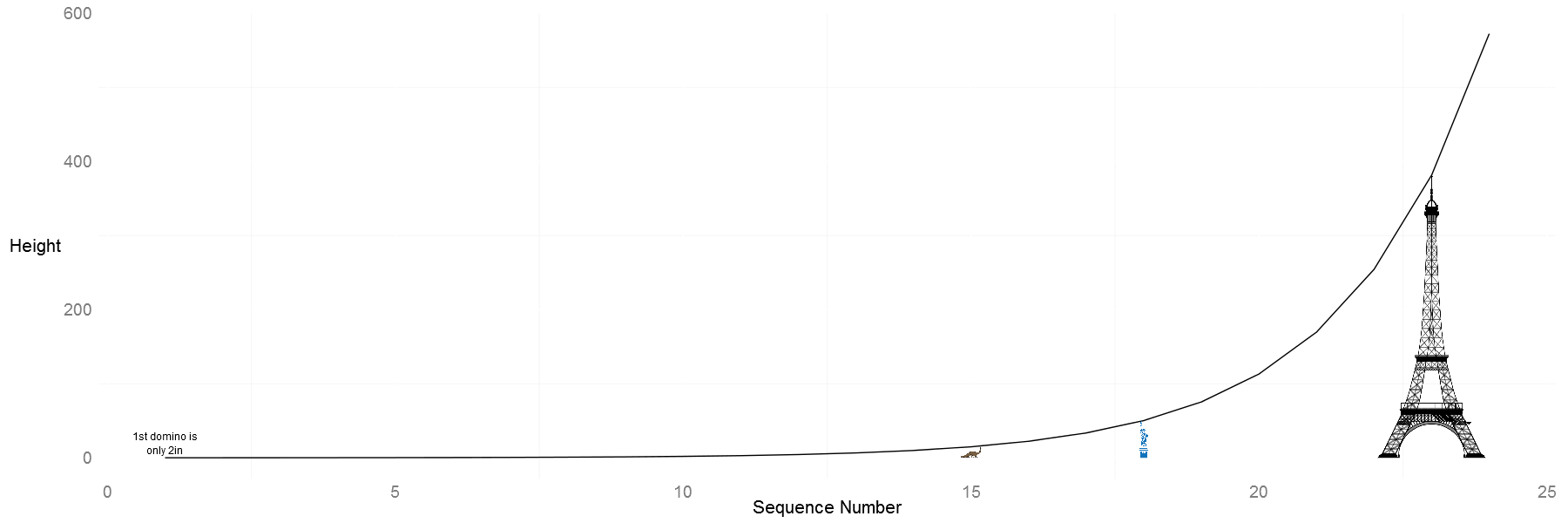Shucks! All this for this boring looking graph. Not lying is not fun. Although the Argentinosaurus, statue of liberty, and Eiffel Tower all are proportionate to their heights, the plot lacks appeal. I thought the next best thing would be to place all the objects close to their values on the x-axis. Another benefit of this approach: I added some other objects that have very small and big y-axis values i.e. a domino, the space station and our moon.

#### Step 5: Place more images using a custom function

```#create a data frame to store file names and x/y coordinates grob_placement <- data.frame(imgname = c("dinosaur-long-neck.png", "statue-of-liberty.png", "eiffel-tower.png", "space-station.png", "moon.png"), xmins = c(13, 17, 20, 38, 53), ymins = rep(-1*10^8, 5), ymaxs = rep(-4.5*10^8, 5), stringsAsFactors = FALSE) grob_placement\$xmaxs <- grob_placement\$xmins + 4   #make a function to create the grobs and call the annotation_custom function add_images <- function(df) { dlply(df, .(imgname), function(df){ img <- readPNG(unique(df\$imgname)) grb <- rasterGrob(img, interpolate = TRUE) annotation_custom(grb, xmin = df\$xmins, xmax = df\$xmax, ymin = df\$ymins, ymax = df\$ymaxs) }) }```

#### Step 6: Add text labels

```#text data frame with x/y coordinates img_texts <- data.frame(imgname = c("domino", "dino", "space-station", "moon"), xs = c(1, 13, 38, 53), ys = rep(-5.2*10^8, 4), texts = c("1st domino is\nonly 2in", "15th domino will reach Argentinosaurus (16m).\nBy 18th domino, you will reach the statue of liberty (46m).\n23 domino will be taller than the Eiffel Tower (300m)", "40th domino will\nreach the ISS (370km)", "57th domino will\nreach the moon (370,000km)" ))   add_texts <- function(df) { dlply(df, .(imgname), function(df){ annotation_custom(grob = textGrob(label = df\$texts, hjust = 0), xmin = df\$xs, xmax = df\$xs, ymin = df\$ys, ymax = df\$ys) }) }```

#### Step 7: Put everything together

```base_plot + add_images(grob_placement) + add_texts(img_texts)   CairoPNG(filename = "domino-effect-geometric-progression-2.png", width = 1800, height = 600, quality = 90) g <- base_plot + add_images(grob_placement) + add_texts(img_texts) + annotation_custom(domino_grob, xmin = 1, xmax = 2, ymin = -1*10^8, ymax = -5*10^8) gt <- ggplot_gtable(ggplot_build(g)) gt\$layout\$clip[gt\$layout\$name == "panel"] <- "off" grid.draw(gt) dev.off()```

This is what we get. Not bad, huh?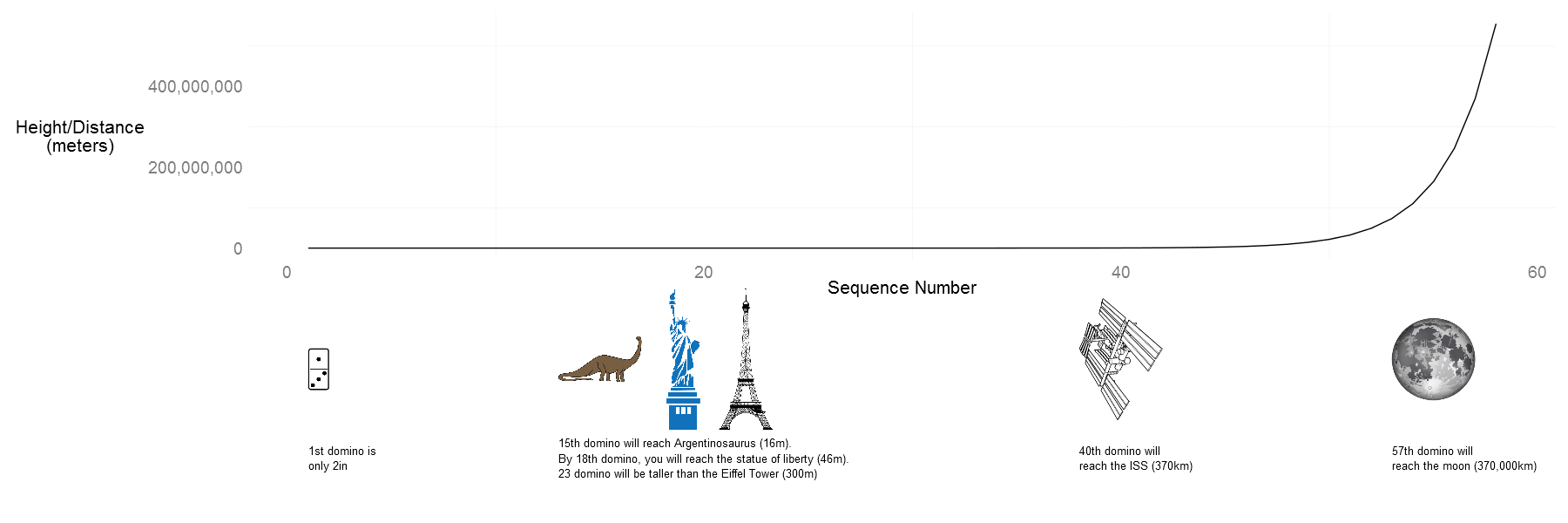We still have a problem: our beloved moon is smaller than the space station, because I placed all the images in rectangles of same height. I could have made the moon slightly bigger, but I could not have maintained the proportion. I thought it is better to have all the objects in similar size rectangles than changing proportions at will. If you have other ideas, please let me know.

#### Step 7: Make it pretty

And by pretty, I mean, upload the final plot to Canva and add the orange color.Here is my final version: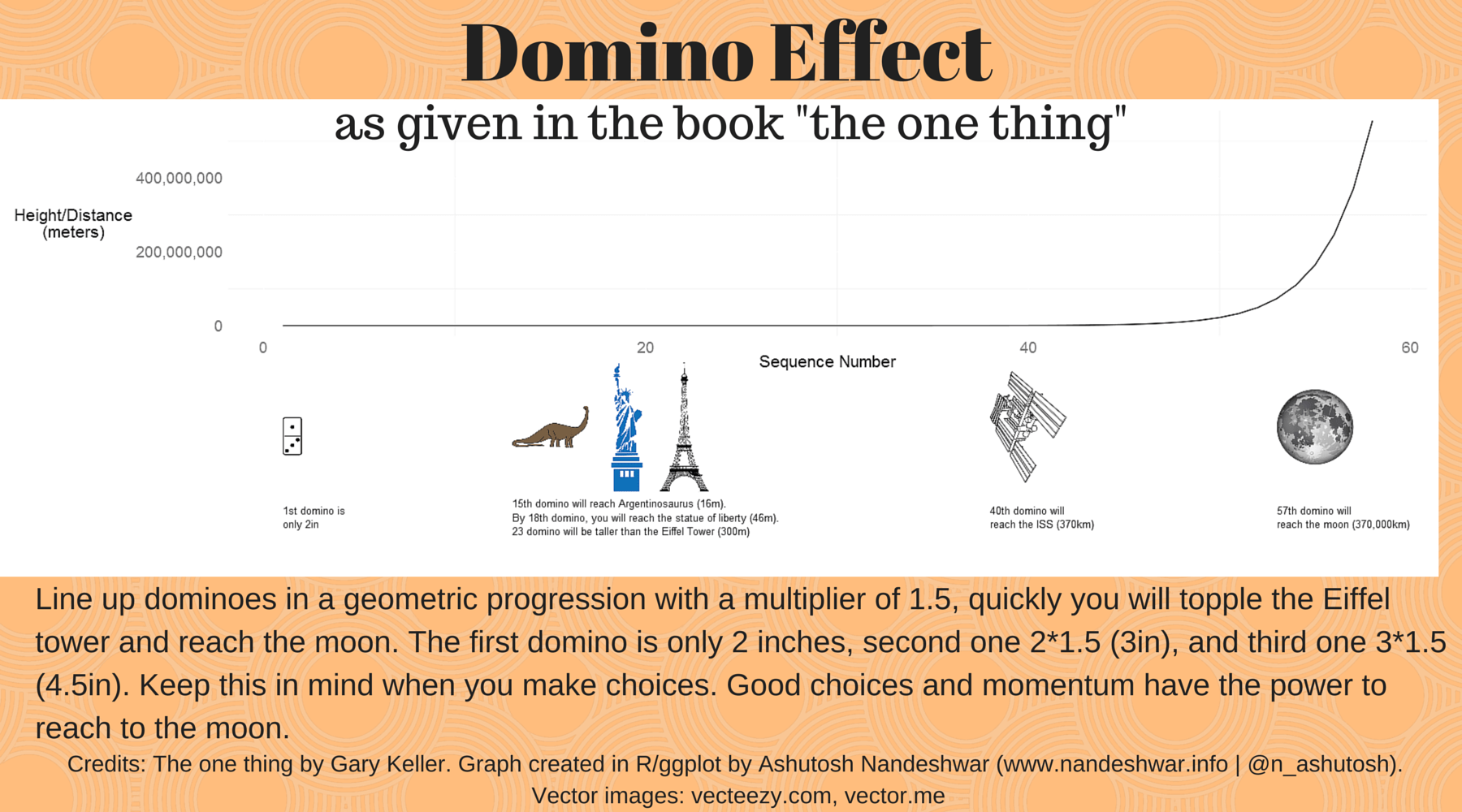There it is! It is possible to use `R` to create infographics or pictograms, and the obvious advantage, as I explained my post Tableau vs. R, is a programming language’s repeatability and reproducibility. You can, of course, edit the output plots in Illustrator or GIMP, but for quick wins, R’s output is fantastic. Can you think of any other ideas to create infographics in R?